# Weierstrass-Erdmann corner conditions

(diff) ← Older revision | Latest revision (diff) | Newer revision → (diff)

Necessary conditions for an extremum, additional to the Euler equation, specified at points at which the extremal has a corner. Let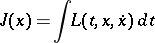be a functional of the classical calculus of variations (cf. Variational calculus), and let the extremal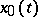be continuously differentiable in a neighbourhood of the pointexcept at the pointitself, at which it has a corner. In this situation, forto be at least a weak local extremum for the functional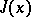, it is necessary that the equations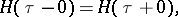where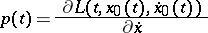and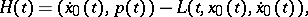be satisfied at the corner point. These equations are known as the corner conditions of K. Weierstrass (1865) and G. Erdmann (1877) .

The meaning of the Weierstrass–Erdmann corner conditions is that the canonical variables and the Hamiltonian are continuous at a corner point of the extremal; their meaning in classical mechanics is the continuity of momentum and of energy at a corner point.

In regular problems, whenis a strictly convex function of, the extremals cannot have corner points. Corner points appear if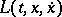and consequently the Weierstrass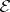-function, contains segments of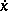. For the Lagrange problem with conditions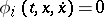and Lagrange multipliers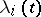, thein the Weierstrass–Erdmann corner conditions is replaced by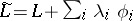.

How to Cite This Entry:
Weierstrass-Erdmann corner conditions. Encyclopedia of Mathematics. URL: http://encyclopediaofmath.org/index.php?title=Weierstrass-Erdmann_corner_conditions&oldid=16105
This article was adapted from an original article by V.M. Tikhomirov (originator), which appeared in Encyclopedia of Mathematics - ISBN 1402006098. See original article